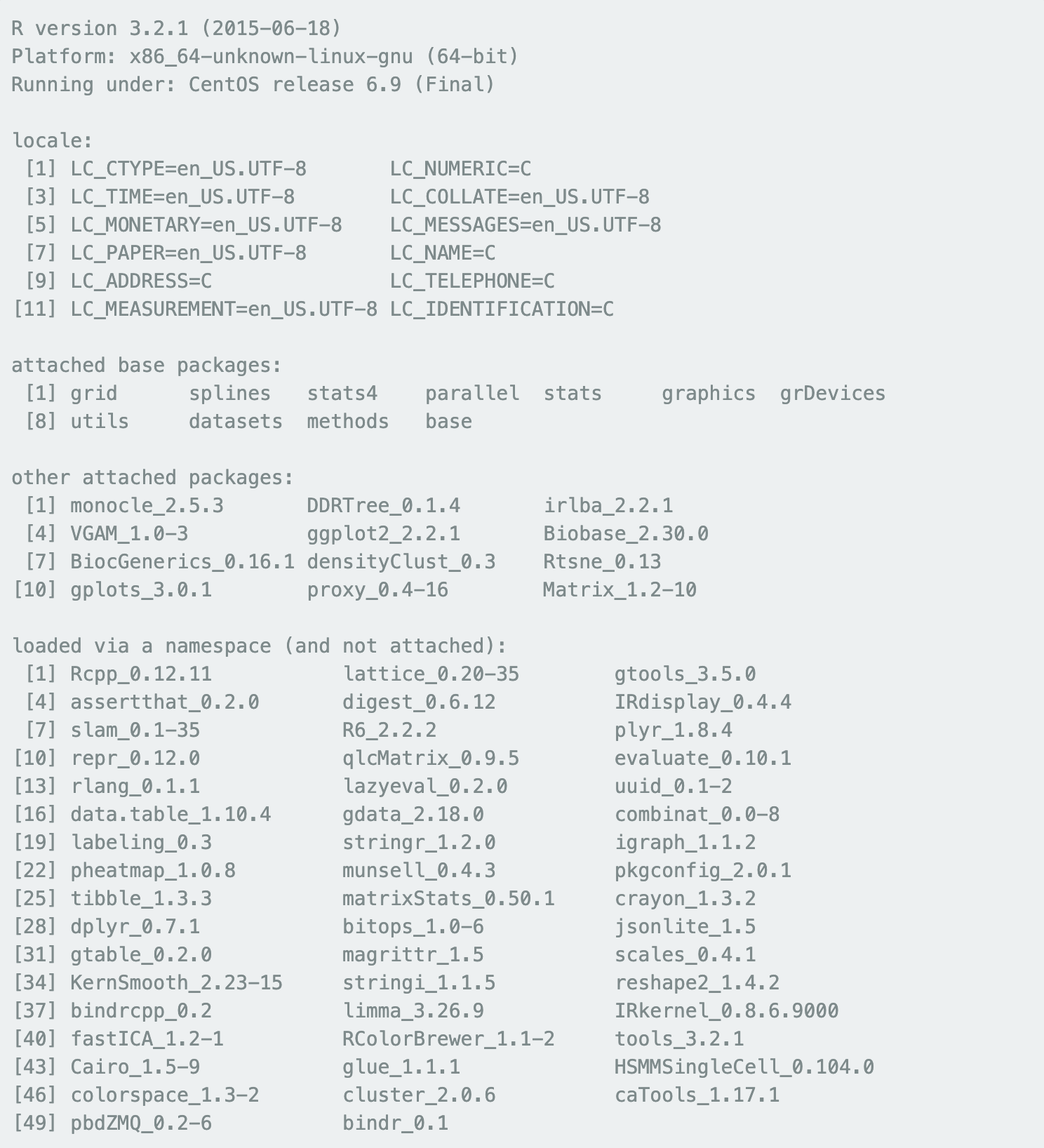Introduction

This notebook will walk you through some of the analyses presented in (Cusanovich et. al (bioRxiv 2017) for working with sci-ATAC-seq data from developing Drosophila melanogaster embryos. The tutorial is broken into the following sections:

2. Using t-SNE to identify finer-scale structure, jump to section re-cluster cells with t-SNE
3. Identifying differentially accessible sites between clusters of cells jump to differential accessibility
4. Arranging cells along developmental trajectories with pseudotemporal ordering, jump to order cells in development

Installation

#### Required R Packages

• Matrix
• proxy
• gplots
• Rtsne
• densityClust from here
• DDRTree (version 0.1.4)
• monocle (version 2.5.3)
• irlba (version 1.0.3)

It is important that the correct version of monocle, DDRTree and irlba are installed. Other versions may produce different results. irlba v1.0.3 was used to generate the figures in the manuscript that are relevant to use cases 1 & 2, while monocle v2.5.3 and DDRTree are the versions that were used for the trajectories presented in the paper. For the first two use cases, we will install the legacy version of irlba (1.0.3) and for the second two we will detach irlba and then load monocle 2.5.3 and DDRTree 0.1.4. To install these packages, open a command line and run:

`curl -O https://cran.r-project.org/src/contrib/Archive/irlba/irlba_1.0.3.tar.gzmkdir ./old_irlbaR CMD INSTALL -l ./old_irlba irlba_1.0.3.tar.gzcurl -O http://krishna.gs.washington.edu/content/members/cusanovich/fly_embryogenesis/updated_data/vignette/monocle_2.5.3.tar.gzR CMD INSTALL monocle_2.5.3.tar.gzcurl -O https://cran.rstudio.com/src/contrib/Archive/DDRTree/DDRTree_0.1.4.tar.gzR CMD INSTALL DDRTree_0.1.4.tar.gz`

` suppressPackageStartupMessages({ library(Matrix) library(proxy) library(gplots) library(Rtsne) library(densityClust) library(irlba,lib.loc="./old_irlba/") }) sessionInfo()`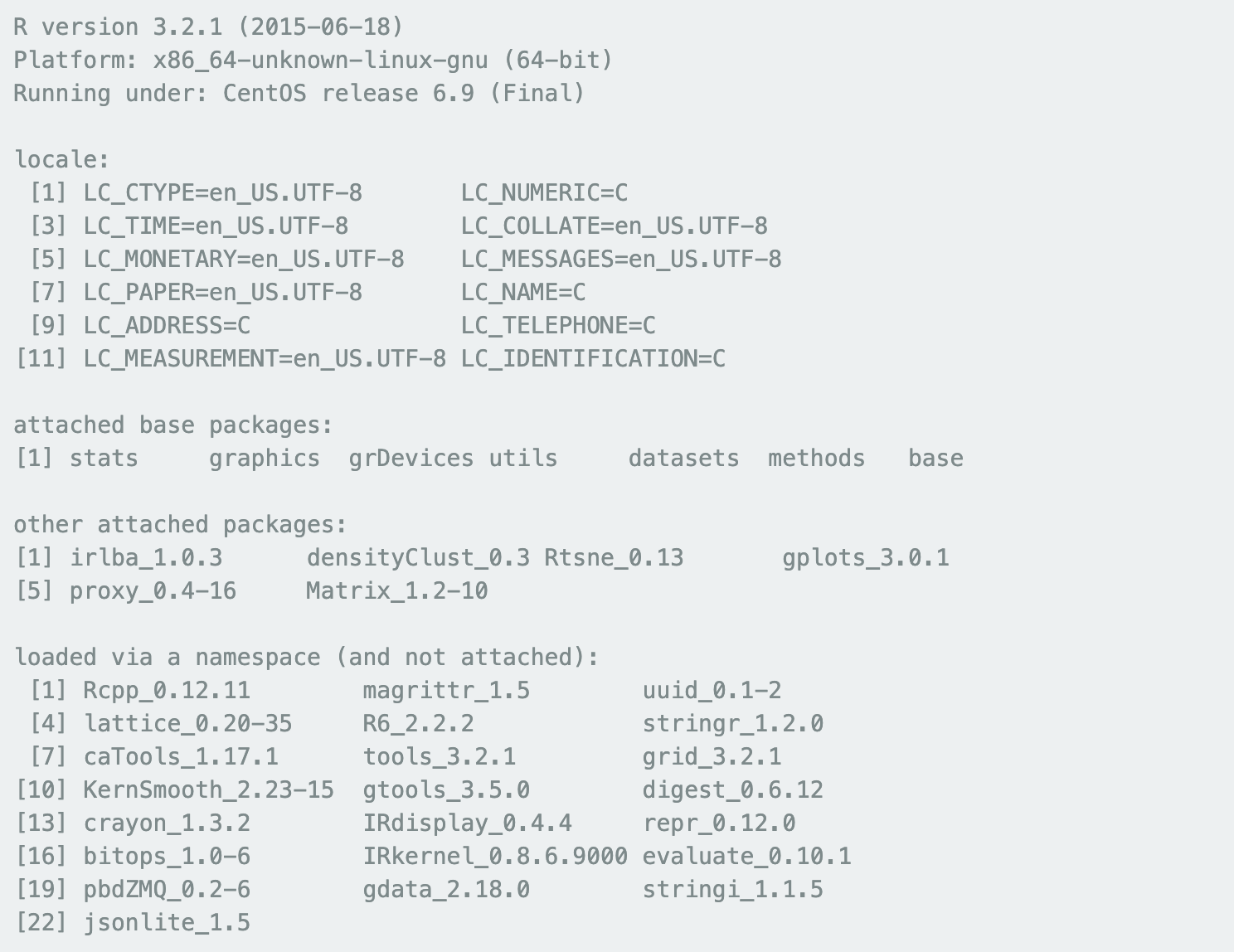Use case 1: ID clades with LSI

We have found it convenient to store the data as a sparse, binary matrix of genomic loci x cells. Below we will walk you through an analysis where individual cells have been scored for insertions in 2kb windows throughout the genome. Note that if you want to go back to the raw sequencing data (FASTQ files), they are available at the Gene Expression Omnibus under accession code GSE101581. We have made tools for manipulating these files available on Github. Alternatively, we have a great number of resources for interacting with the data at our companion site.

` download.file( "http://krishna.gs.washington.edu/content/members/cusanovich/fly_embryogenesis/updated_data/vignette/6to8.2kbmatrix.sparse.binary.rds", destfile = "6to8.2kbmatrix.sparse.binary.rds") flies_6to8 = readRDS("6to8.2kbmatrix.sparse.binary.rds")`

After filtering out 2kb windows that intersect with ENCODE-defined blacklist regions, we end up with 83,290 distinct 2kb windows in the drosophila genome and we have data from 7,880 cells at the 6-8 hour time point.

`dim(flies_6to8) flies_6to8[1:2,1:2]`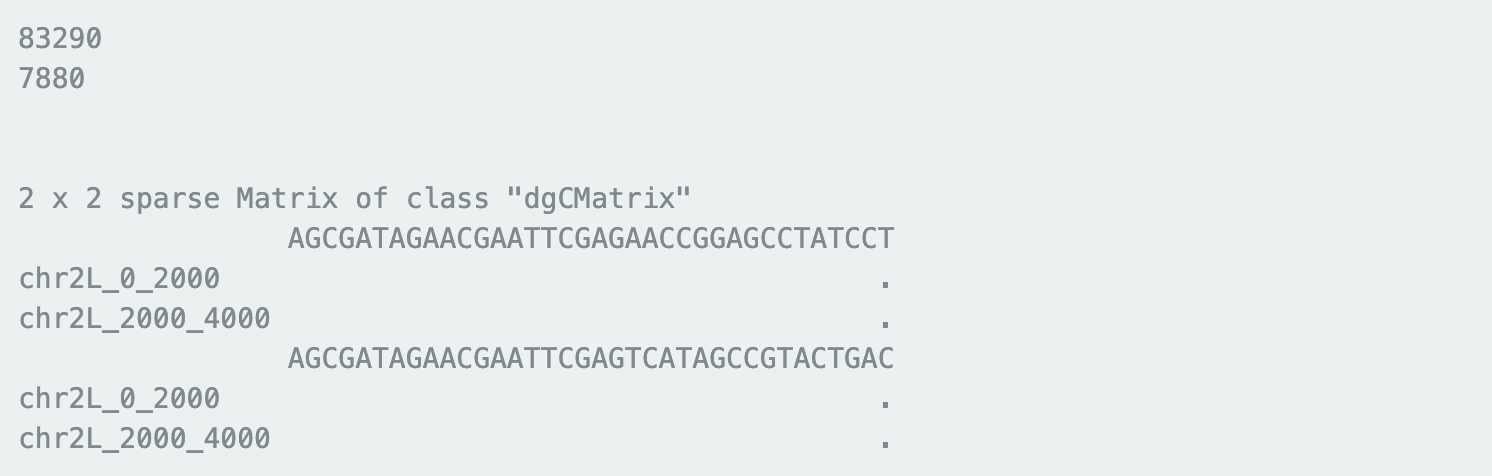Let’s collect some info about the frequency of insertion in individual windows and the variety of windows observed in individual cells. We can see that sites are observed in as many as 6,322 cells (80%), but are roughly normally distributed (with a long lower tail) on a log scale with a median of 330 cells having an insertion in each site.

`num_cells_ncounted = rowSums(flies_6to8) options(repr.plot.width=4, repr.plot.height=4) hist(log10(num_cells_ncounted),main="No. of Cells Each Site is Observed In",breaks=50) abline(v=log10(num_cells_ncounted[order(num_cells_ncounted,decreasing=T)]),lwd=2,col="indianred")`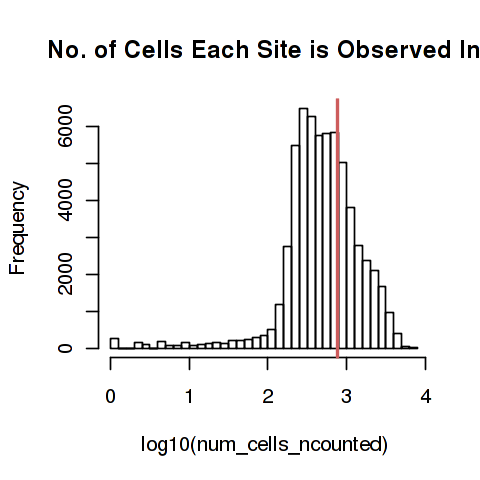Now let us only retain the most commonly used sites (top 20,000 here). Looking at the distribution of how many of these top 20,000 sites each cell covers, we can again see that the distribution is rougly log-normal (with a long lower tail) with a median of 3,751 windows covered by each cell.

`ncounts = flies_6to8[which(num_cells_ncounted >= num_cells_ncounted[order(num_cells_ncounted,decreasing=T)]),] new_counts = colSums(ncounts) hist(log10(new_counts),main="Number of Sites Each Cell Uses",breaks=50) abline(v=log10(quantile(new_counts,probs=0.1)),lwd=2,col="indianred")`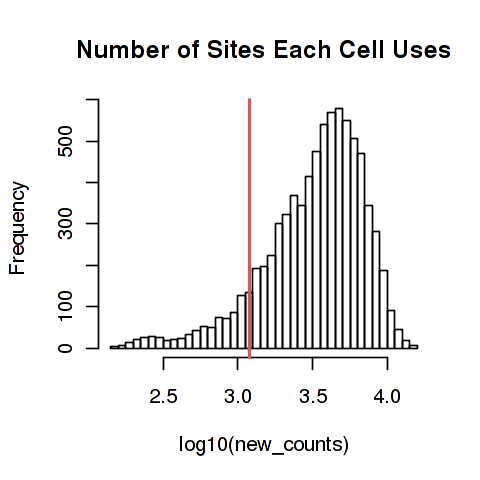Before transforming the data, we just filter out the lowest 10% of cells (in terms of site coverage) and ensure that there are now empty sites.

` ncounts = ncounts[,new_counts >= quantile(new_counts,probs=0.1)] ncounts = ncounts[rowSums(ncounts) > 0,]`

We can now transform the data using TF-IDF and then generate a lower dimensional representation of the data with truncated SVD (these are the two primary steps of LSI).

`nfreqs = t(t(ncounts) / Matrix::colSums(ncounts)) idf = as(log(1 + ncol(ncounts) / Matrix::rowSums(ncounts)), "sparseVector") tf_idf_counts = as(Diagonal(x=as.vector(idf)), "sparseMatrix") %*% nfreqs`

Here, we only retain components 2-6 (component 1 is highly correlated with read depth) and truncate the distribution of LSI values at +/-1.5.

`#This step can take a minute set.seed(0) #For reproducibility SVD = irlba(tf_idf_counts, 6, 6) sk_diag = matrix(0, nrow=6, ncol=6) diag(sk_diag) = SVD\$d sk_diag[1,1] = 0`

`LSI_out = t(t(sk_diag %*% t(SVD\$v)) %*% t(SVD\$u)) LSI_out = t(scale(t(LSI_out))) LSI_out[LSI_out > 1.5] = 1.5 LSI_out[LSI_out < -1.5] = -1.5 `

Next, we generate some info about the dendrograms that will be used for plotting the heatmap.

` #This step can take a minute too hclust_cells = hclust(proxy::dist(t(sk_diag %*% t(SVD\$v)), method="cosine"), method="ward.D2") hclust_genes = hclust(proxy::dist(t(sk_diag %*% t(SVD\$u)), method="cosine"), method="ward.D2") ` ` color_pal = c("#1F78B4","#FFD700","#60CC52","#E31A1C") hmcols = colorpanel(100, "steelblue", "white", "tomato") cells_tree_cut = cutree(hclust_cells, 4) lsi_cells = cbind(colnames(ncounts),cells_tree_cut) `

Finally, we can generate a bi-clustered heatmap showing how cells and sites are related.

` options(repr.plot.width=4, repr.plot.height=6) heatmap.2(LSI_out, col=hmcols, ColSideColors=color_pal[as.factor(cells_tree_cut)], #RowSideColors=color_pal[as.factor(genes_tree_cut)], Rowv = as.dendrogram(hclust_genes), Colv = as.dendrogram(hclust_cells), labRow=FALSE, labCol=FALSE, trace="none", scale="none", useRaster=TRUE) `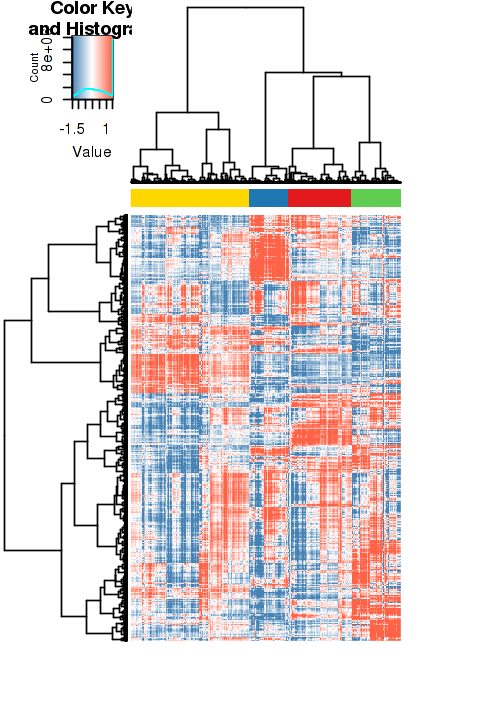` rm(flies_6to8) rm(ncounts) rm(nfreqs) rm(tf_idf_counts) rm(SVD) rm(LSI_out) `

Use case 2: Re-cluster cells with t-SNE

Having identified large clades of cells that were consistent with the development of germ layers during embryogenesis, we were able to identify peaks of chromatin accessibility in each clade after “in silico sorting” the cells assigned to each cluster. We next sought to robustly identify smaller clusters of cells that might be more consistent with individual cell types so that we could learn about the individual regulatory elements that govern distinct cell states. To do so, we generated a master list of summits of accessibiity identified in each of the clades across the three time points and then created a matrix of sites by cells (similar to the last use case).

` download.file( "http://krishna.gs.washington.edu/content/members/cusanovich/fly_embryogenesis/updated_data/vignette/6to8.summitmatrix.sparse.binary.rds", destfile = "6to8.summitmatrix.sparse.binary.rds") fly_summits_6to8 = readRDS("6to8.summitmatrix.sparse.binary.rds") dim(fly_summits_6to8) fly_summits_6to8[1:2,1:2] `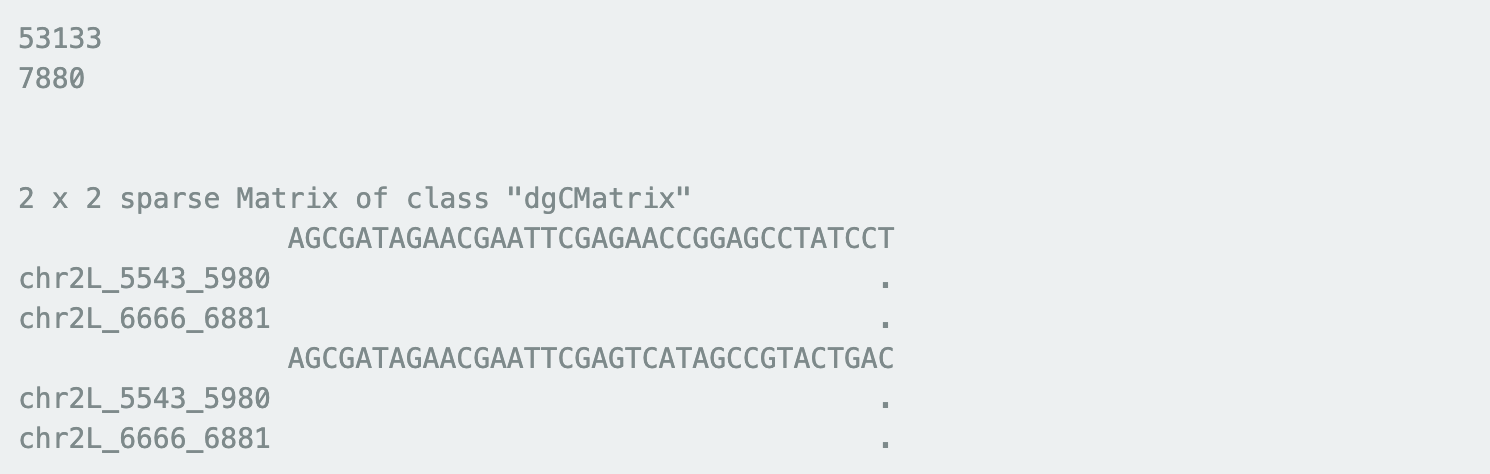The analysis starts out similarly to the LSI example above. We first filter out sites that are seen in fewer cells (in this case we only keep sites that are seen in at least 5% of cells) and then cells that have relatively low coverage (again, we filter out the lowest 10% of cells).

` num_cells_ncounted = rowSums(fly_summits_6to8) ncounts = fly_summits_6to8[num_cells_ncounted >= dim(fly_summits_6to8)*0.05,] new_counts = colSums(ncounts) ncounts = ncounts[,new_counts >= quantile(new_counts,probs=0.1)] ncounts = ncounts[rowSums(ncounts) > 0,] `

` options(repr.plot.width=4, repr.plot.height=4) hist(log10(num_cells_ncounted),main="No. of Cells Each Site is Observed In",breaks=50) abline(v=log10(min(num_cells_ncounted[num_cells_ncounted >= dim(fly_summits_6to8)*0.05])),lwd=2,col="indianred") hist(log10(new_counts),main="Number of Sites Each Cell Uses",breaks=50) abline(v=log10(quantile(new_counts,probs=0.1)),lwd=2,col="indianred") `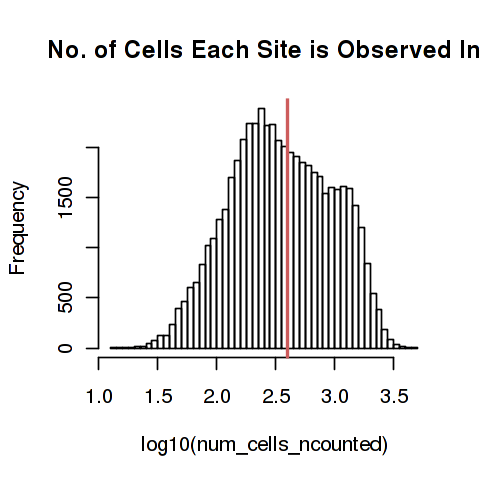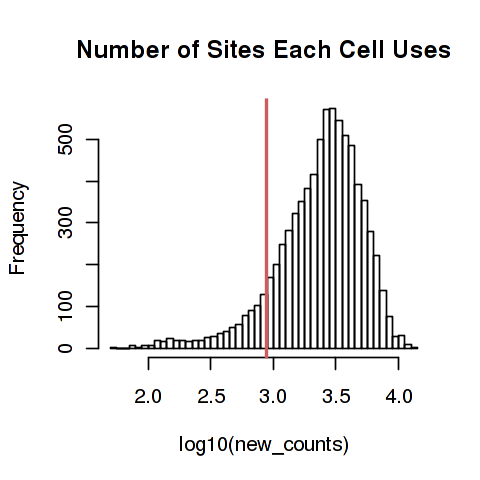The next step is to tranform the data and generate a lower dimensional representation again, except that we first filter out sex chromosome counts. We also leave the first component in now, and we use 50 dimensions (rather than 6).

` sexsites = c(grep("chrY",rownames(ncounts)),grep("chrX",rownames(ncounts))) ncounts.nosex = ncounts[-sexsites,] `

` nfreqs = t(t(ncounts.nosex) / Matrix::colSums(ncounts.nosex)) idf = as(log(1 + ncol(ncounts.nosex) / Matrix::rowSums(ncounts.nosex)), "sparseVector") tf_idf_counts = as(Diagonal(x=as.vector(idf)), "sparseMatrix") %*% nfreqs `

` set.seed(0) SVDtsne = irlba(tf_idf_counts, 50, 50) d_diagtsne = matrix(0, nrow=50, ncol=50) diag(d_diagtsne) = SVDtsne\$d SVDtsne_vd = t(d_diagtsne %*% t(SVDtsne\$v)) `

` dim(ncounts.nosex) ncounts.nosex[1:2,1:2] tf_idf_counts[1:2,1:2] `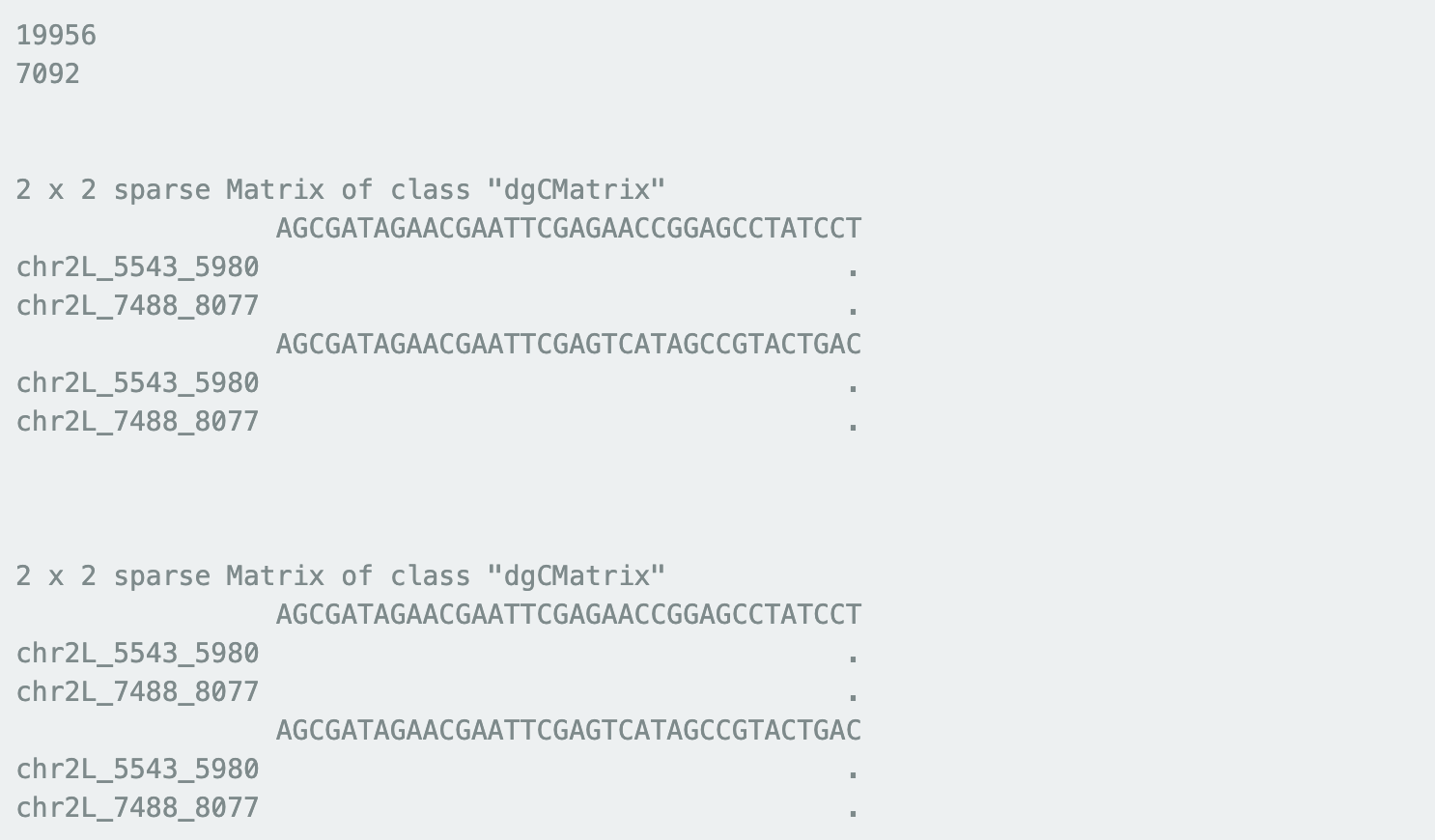Next, we use t-SNE to visualize the data. We feed this lower dimensional representation of the data directly into the Rtsne package.

` set.seed(0) `
` tsnetfidf = Rtsne(SVDtsne_vd,pca=F) `

To identify clusters of cells, we use the density peak algorithm.

`tsnedist = dist(tsnetfidf\$Y) `
` set.seed(0) `
` dclust = densityClust(tsnedist,gaussian=T) `
` dclust = findClusters(dclust, rho = 50, delta = 2.5) `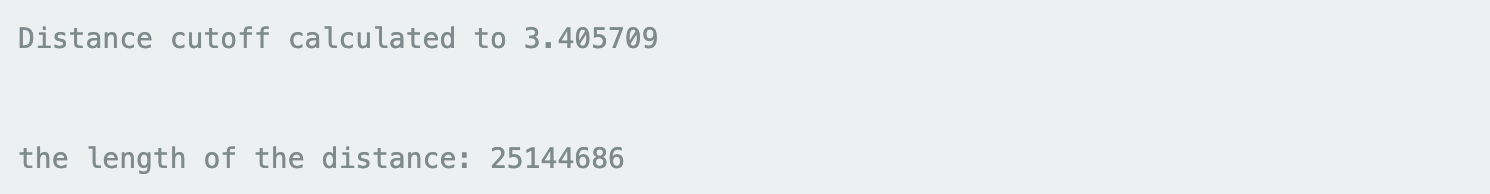The density peak algorithm requires you to set two parameters delta and rho. For each data point, the algorithm calculates a local density of other points within some set distance and the minimum distance to the next point that has a higher local density. On the basis of these two values, you can choose a set of points that are outliers both in local density and the distance to another point with a higher density, which become cluster peaks. Below, we show you the distribution of these two values in our data set and where we decided to draw the cutoff. You can read more about this algorithm here.

` options(repr.plot.width=6, repr.plot.height=6) plot(dclust\$rho,dclust\$delta,pch=20,cex=0.6) points(dclust\$rho[dclust\$peaks],dclust\$delta[dclust\$peaks],col="red",pch=20,cex=0.8) text(dclust\$rho[dclust\$peaks]-2,dclust\$delta[dclust\$peaks]+1.5,labels=dclust\$clusters[dclust\$peaks]) abline(v=50) abline(h=2.5) `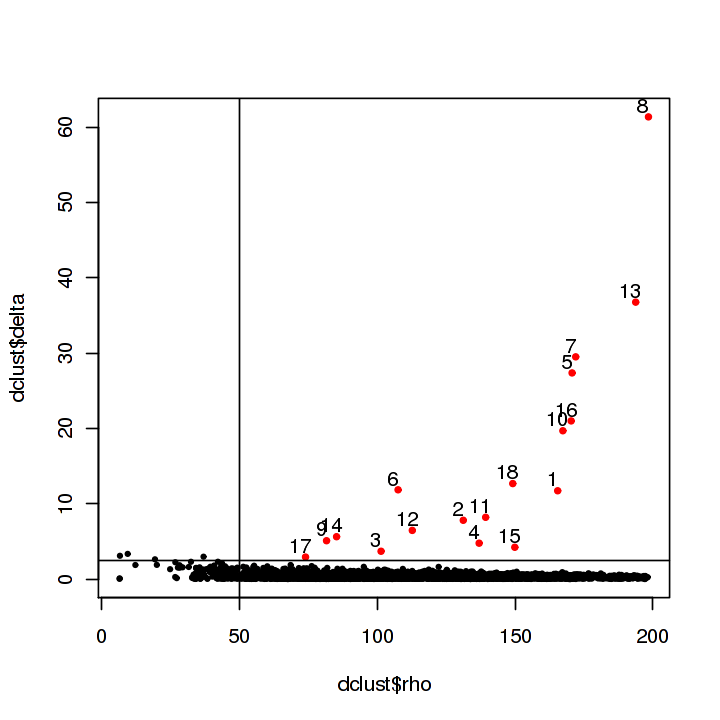Finally, we plot the t-SNE plots and show how the points are related to our original LSI clustering, the assigned sex of each cell (you will need to download an additional file for this), and curren density peak clusters.

` download.file( "http://krishna.gs.washington.edu/content/members/cusanovich/fly_embryogenesis/updated_data/vignette/6to8.xycalls.txt", destfile = "6to8.xycalls.txt") sexcolors = read.table("6to8.xycalls.txt") `

` tsnecols = c("#E31A1C","#FFD700","#771122","#777711","#1F78B4","#68228B","#AAAA44", "#60CC52","#771155","#DDDD77","#774411","#AA7744","#AA4455","#117744", "#000080","#44AA77","#AA4488","#DDAA77") options(repr.plot.width=8, repr.plot.height=8) par(mfrow=c(2,2)) plot(tsnetfidf\$Y,pch=20,main="No Clusters",cex=0.25) plot(tsnetfidf\$Y,pch=20, col=c("#1F78B4","#FFD700","#60CC52","#E31A1C")[as.factor(lsi_cells[match(colnames(tf_idf_counts),lsi_cells[,1]),2])], main="LSI Clusters",cex=0.25) plot(tsnetfidf\$Y,pch=20,col=c("blue","red")[sexcolors[match(colnames(tf_idf_counts),sexcolors[,1]),2]], main="M/F Coloring",cex=0.25) plot(tsnetfidf\$Y,pch=20,col=tsnecols[as.factor(dclust\$clusters)],main="Density Peak Clusters",cex=0.25) text(tsnetfidf\$Y[dclust\$peaks,1],tsnetfidf\$Y[dclust\$peaks,2],labels=dclust\$clusters[dclust\$peaks],cex=2.5) `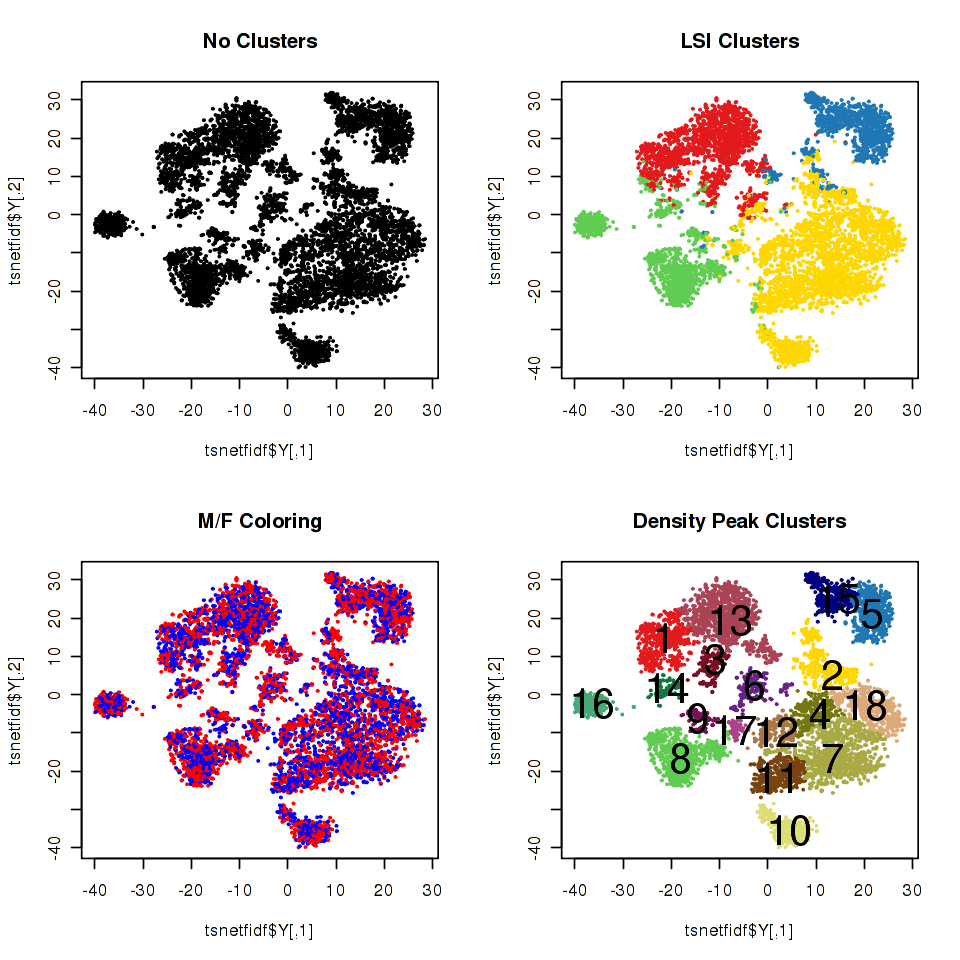` rm(fly_summits_6to8) rm(ncounts) rm(ncounts.nosex) rm(nfreqs) rm(tf_idf_counts) rm(SVDtsne) gc() `

Use case 3: Differential accessibility

Having defined clusters of cells on the basis of chromatin accessibility profiles, you might like to know which sites in the genome are different between clusters. To figure that out, we use the linear modelling framework implemented in Monocle. This use case will walk you through the steps to define which sites are open in mesodermal cells relative to all other cells in the time point, but the same framework could be applied to any comparison you like.

Before getting into the analysis, we need to take care of a few housekeeping things. First, we want to load Monocle to run the differential accessibility tests. To do this we detach the legacy version of irlba and then load Monocle. Finally, we created a little patch to Monocle that reports beta values from the differential accessibility tests so that we can distinguish sites that are opening from sites that are closing.

NOTE: This code is implemented to run on multiple processors on a computing cluster, you may need special modifications to get this working in your preferred computing environment.

` suppressPackageStartupMessages({ detach("package:irlba", unload=TRUE) library(monocle) }) download.file( "http://krishna.gs.washington.edu/content/members/cusanovich/fly_embryogenesis/updated_data/vignette/monocle_patch.R", destfile = "monocle_patch.R") source("monocle_patch.R") download.file( "http://krishna.gs.washington.edu/content/members/cusanovich/fly_embryogenesis/updated_data/vignette/6to8.read.report.txt", destfile = "6to8.read.report.txt") readcounts = read.table("6to8.read.report.txt",header=T) `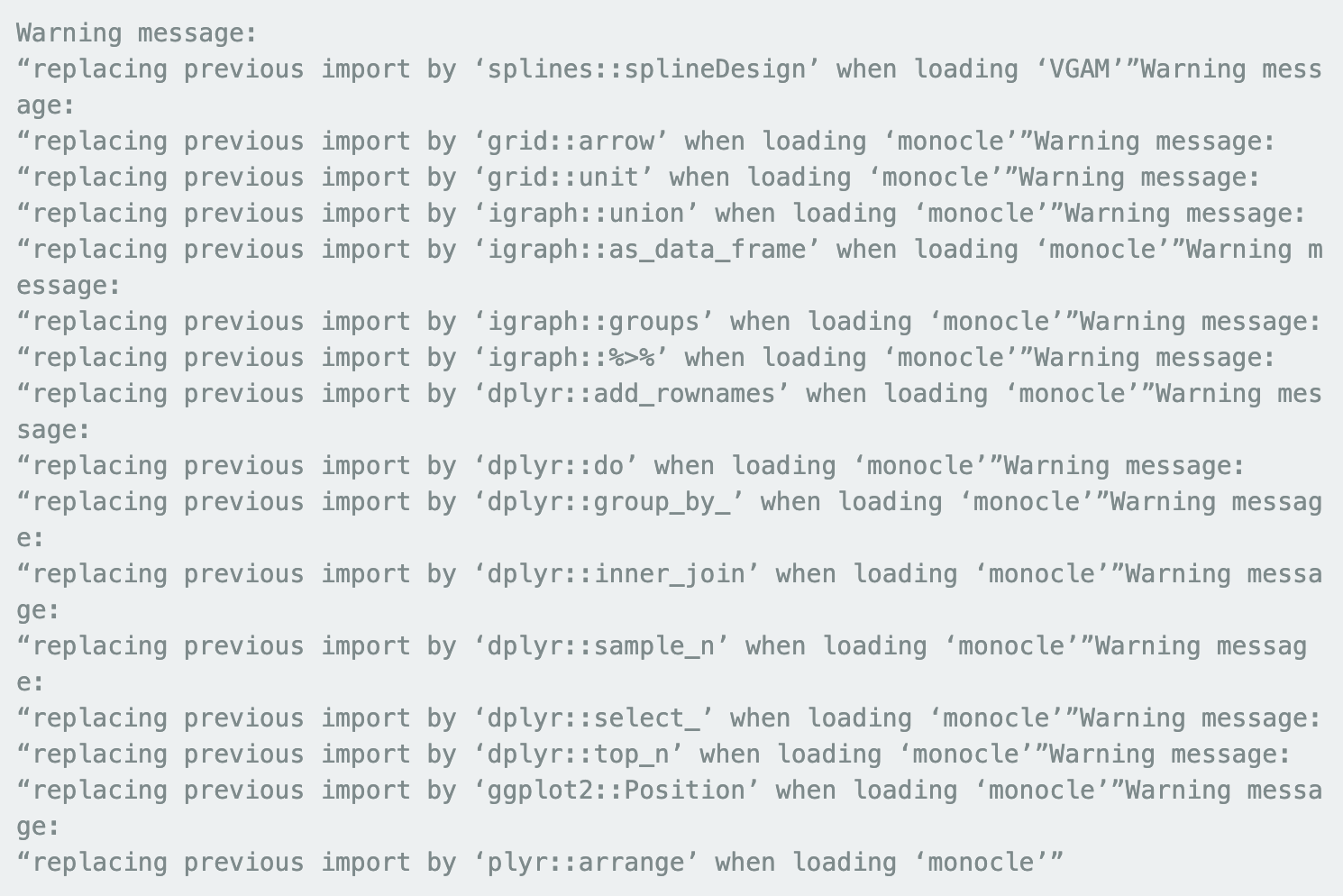Because the summit matrix includes data for more cells, we first subset it cells that we have germ layer predictions for. We then filter out any sites that were not observed in at least 50 cells.

`goodcells = match(lsi_cells[,1],colnames(fly_summits_6to8))da_mat = fly_summits_6to8[,goodcells]num_cells_ncounted = rowSums(da_mat)da_mat_final = da_mat[num_cells_ncounted >= 50,]`

`rm(da_mat)gc()`

 used (Mb) gc trigger (Mb) max used (Mb) Ncells 1747068 93.4 11554252 617.1 14442815 771.4 Vcells 443259864 3381.9 2642652780 20161.9 3302654927 25197.3

We next want to load our site x cell matrix into a Cell Data Set (CDS), a format that allows us to use some of the convenience functions available in monocle. The first step is to set up a phenotype data frame. This is a framework for storing all kinds of information about individual cells.

`readcounts.assigned = readcounts[match(lsi_cells[,1],rownames(readcounts)),]clusterfactor = factor((lsi_cells[,2] == '4')+0,levels=c("0","1"))pda = data.frame(as.character(lsi_cells[,1]),as.numeric(log10(readcounts.assigned[,2])),clusterfactor)names(pda) = c("CellID", "ReadDepth","CellCluster")pda = new("AnnotatedDataFrame", data = pda)`

We next set up a "feature" data frame - a framework for storing information about data features (in this case, sites).

`fda = as.data.frame(as.character(rownames(da_mat_final)))names(fda) = "Peak"fda = new("AnnotatedDataFrame", data = fda)`

And then we can combine the feature data frame and phenotype data frame with the raw site x cell matrix into a CDS. Because the data are binary calls of insertions in sites, we set the `expressionFamily` to binomialff.

`rownames(da_mat_final) = NULLcolnames(da_mat_final) = NULLsubmat_cds = newCellDataSet(da_mat_final,featureData = fda,phenoData = pda,expressionFamily=binomialff(),lowerDetectionLimit=1)`

`pData(submat_cds)\$Size_Factor = 1`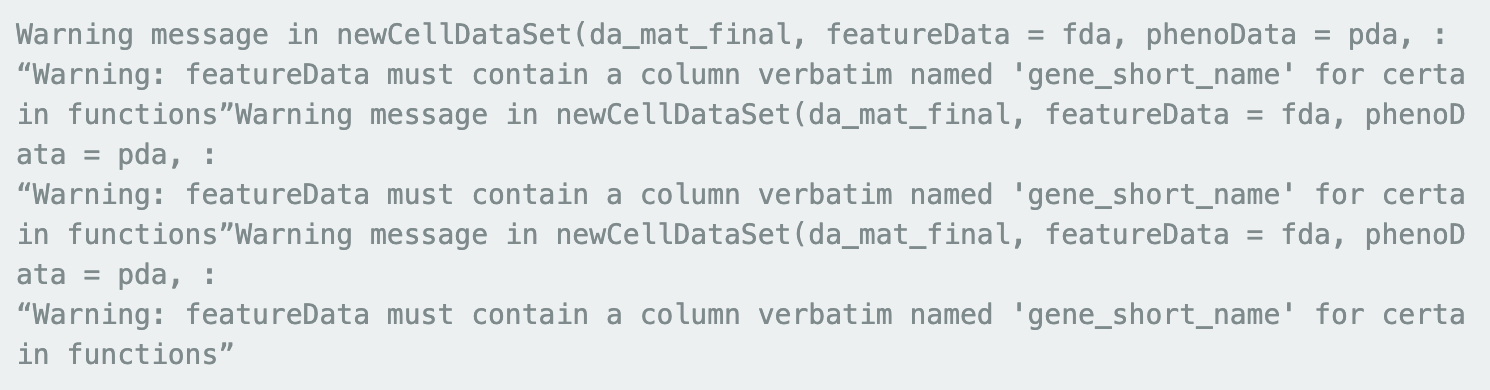Here we just delete some variables that we will not need anymore to free up RAM.

`rm(readcounts.assigned)rm(fda)rm(pda)rm(da_mat_final)gc()`

 used (Mb) gc trigger (Mb) max used (Mb) Ncells 1810190 96.7 9243401 493.7 14442815 771.4 Vcells 443430760 3383.2 2114122224 16129.5 3302654927 25197.3

And now we re ready to run the likelihood ratio tests to identify differentially accessible sites. Monocle provides a convenient wrapper for doing this with the `differentialGeneTest` function. This step can take several minutes to run.

` differtest = differentialGeneTest(submat_cds, fullModelFormulaStr = "~CellCluster + ReadDepth", reducedModelFormulaStr = "~ReadDepth", cores=15) head(differtest[order(differtest\$qval),])`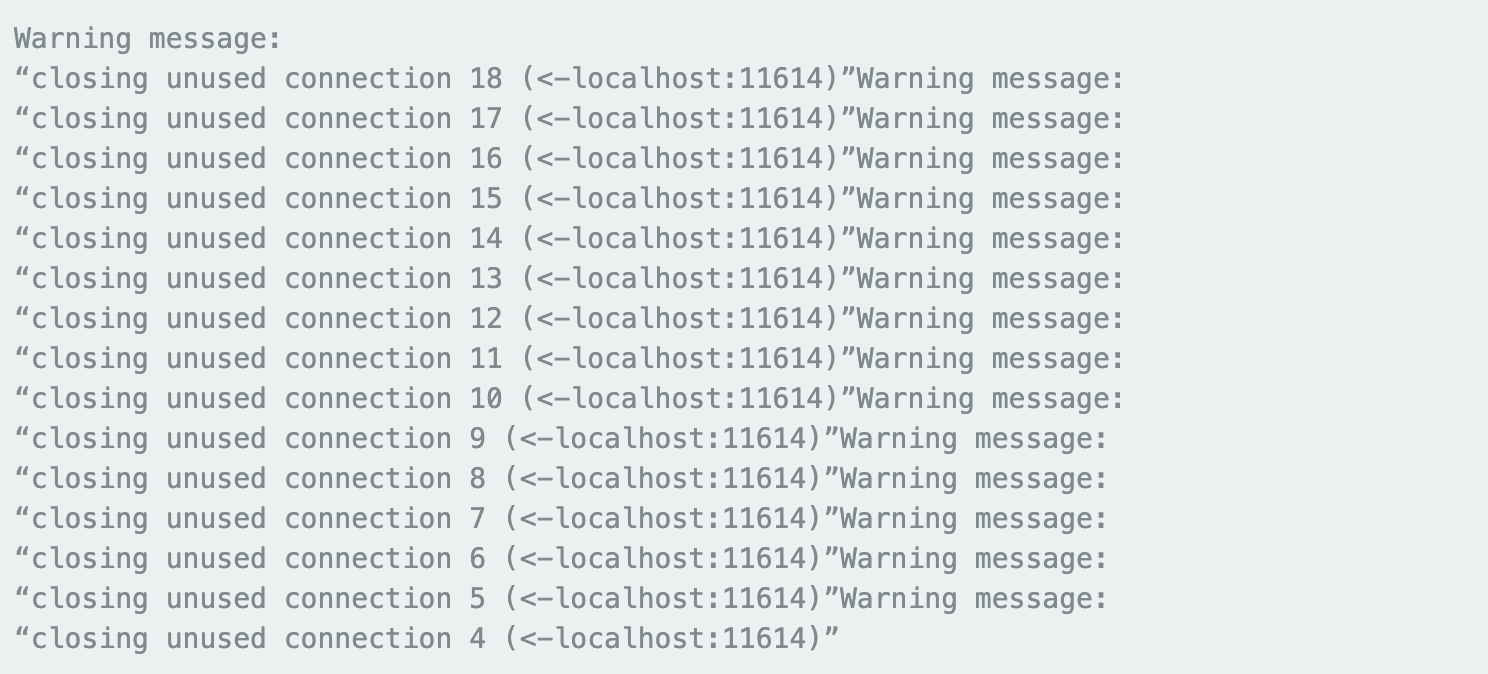status family pval beta qval Peak 24326 OK binomialff 0.000000e+00 2.778820 0.000000e+00 chr3L_10547945_10548368 38943 OK binomialff 0.000000e+00 3.194512 0.000000e+00 chr3R_18852360_18852769 8709 OK binomialff 0.000000e+00 3.442576 0.000000e+00 chr2L_20476729_20477328 8716 OK binomialff 1.388543e-311 3.344137 1.809584e-307 chr2L_20486338_20486627 12015 OK binomialff 1.716059e-305 3.306066 1.789129e-301 chr2R_5822850_5823121 19994 OK binomialff 4.726260e-298 2.672527 4.106254e-294 chr3L_589563_589900

With this test, we find 19,535 sites are differentially accessible (1% FDR) in myogenic mesoderm cells relative to all other cells in the embryo. 8,398 of these are more accessible in myogenic mesoderm than other cells, while 11,137 are less accessible in myogenic mesoderm. We can see that the sites in the top of the list are consistent with what you would expect (for example, the second most significant hit happens to be the promoter of lmd, a known master regulator of fusion-competent myoblasts.

` options(repr.plot.width=8, repr.plot.height=4) par(mfrow=c(1,2)) plot(tsnetfidf\$Y,pch=20, col=c("#1F78B4", "#FFD700", "#60CC52", "#E31A1C")[as.factor(lsi_cells[match(colnames(tf_idf_counts), lsi_cells[,1]),2])],main="LSI Clusters",cex=0.25,xlab="t-SNE1",ylab="t-SNE2") plot(tsnetfidf\$Y[match(pData(submat_cds)\$CellID,colnames(tf_idf_counts)),][order(exprs(submat_cds)[38943,]),],pch=20, main="lmd Promoter+ Cells",cex=0.25, col=ifelse(exprs(submat_cds)[38943,][order(exprs(submat_cds)[38943,])] > 0,"#E31A1C","gray"), xlab="t-SNE1",ylab="t-SNE2")`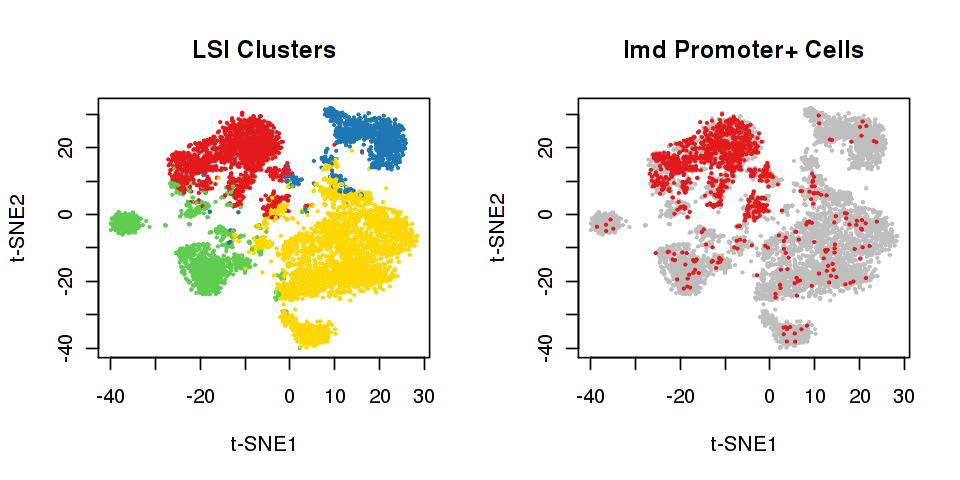` rm(da_mat_final)`
` rm(submat_cds)`
` gc()`

Use case 4: Order cells in development

One very powerful aspect of single-cell technologies is that we can use them to trace the developmental trajectories of cells. In the Cusanovich et al. paper, we showed that sci-ATAC-seq data can be used to identify developmental trajectories. Here we walk you through the basic steps of that analysis.

For this analysis, we turn to the 2-4 hour time point, so we will load a cds for cells from that time point. On the basis of the t-SNE analysis of these cells we were able to classify the cells into the following 7 cell types: Unknown, Collisions, Blastoderm, Neural, Ectoderm, Mesoderm, Endoderm. However, we really wanted to see how cells transitioned from the blastoderm state to the germ layers, so we used monocle to arrange cells in a progression to learn about how the regulatory landscape of the genome changed through development.

One note: instead of using the counts of reads mapping to individual sites of accessibility for this analysis, we binned all the sites that were near eachother in order to improve the sparsity of the data.

To run through this section, we need several files, so we will download a tarball and unpack it before proceeding.

` download.file( "http://krishna.gs.washington.edu/content/members/cusanovich/fly_embryogenesis/updated_data/vignette/2to4_files.tar.gz", destfile = "2to4_files.tar.gz") system("tar -xzf 2to4_files.tar.gz") cds_2to4 = readRDS("./2to4_files/cds_2to4_aggregated.rds") overlapped_sites = read.table('./2to4_files/2to4.overlapped_sites.bed') cell_classification = read.table('./2to4_files/2to4.germlayers.txt') table(cell_classification\$V2)`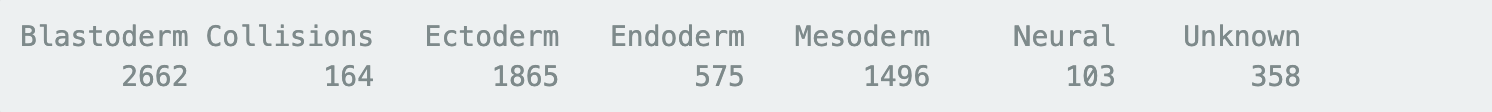After unpacking all those files, we want to collect the top 100 DA sites for each of the cell types found in this time point (excluding sites from the 'Collisions' and 'Unknown' categories). After collecting those sites we need to determine which binned sites they overlap.

` DA_monocle_list = lapply(seq(1,7,1), FUN = function(x) { DA_file_name = paste0('./2to4_files/2to4.sigopen.cluster', x, '.txt') DA_file = read.csv(DA_file_name, sep = ' ', header = F) DA_file\$GL = x return (DA_file) })`

` DA_monocle = data.table::rbindlist(DA_monocle_list) colnames(DA_monocle) = c('chr','start', 'end', 'qvalue', 'GL') DA_monocle\$coord = paste(DA_monocle\$chr, DA_monocle\$start, DA_monocle\$end, sep = '_')`

` get_top_DA = function(cluster = 1, DA_results=DA_GL, num = 500){ DA_this_cluster = subset(DA_results, GL == cluster) DA_this_cluster = DA_this_cluster[order(DA_this_cluster[,qvalue]),] if (num > nrow(DA_this_cluster)){ return (DA_this_cluster[c(1:nrow(DA_this_cluster)),]\$coord) }else {return (DA_this_cluster[c(1:num),]\$coord)} }`

` DA_sites_to_order = unlist(lapply(c(1,3,4,5,6), get_top_DA, DA_results = DA_monocle,num=100)) overlapped_DA_sites_to_order = subset(overlapped_sites, V4 %in% DA_sites_to_order)\$V8`

We then update the 2-4 hour CDS to track what the germ layer assingments are and to establish which sites should be used for ordering cells (the top DA sites we defined above).

` rownames(cell_classification) = cell_classification\$V1 germ_layer_pallet = c('Unknown'='#68228B', 'Blastoderm'='#808080', 'Neural'='#1F78B4', 'Ectoderm'='#FFD700', 'Mesoderm'= '#E31A1C', 'Endoderm'='#60CC52', 'Collisions'='saddlebrown') cell_classification = cell_classification[rownames(pData(cds_2to4)),] cds_2to4\$germ_layer_name = cell_classification\$V2 fData(cds_2to4)\$use_for_ordering = FALSE cds_2to4 = setOrderingFilter(cds_2to4, overlapped_DA_sites_to_order)`

Having set up the CDS, now we use `DDRTree` to learn the developmental trajectory and then arrange cells on that trajectory with the `oderCells` function.

` cds_2to4 = reduceDimension(cds_2to4,reduction_method="DDRTree", ncenter=200, max_components = 3, maxIter=1000, auto_param_selection = FALSE, residualModelFormulaStr="~as.numeric(num_genes_expressed)", )` ` cds_2to4 = orderCells(cds_2to4)`

` #NOTE: This is just a convenience function for plotting to figures side-by-side, since ggplot2 can not do that by default. from http://www.cookbook-r.com/Graphs/Multiple_graphs_on_one_page_(ggplot2) `

` multiplot <- function(..., plotlist=NULL, file, cols=1, layout=NULL) { library(grid) plots <- c(list(...), plotlist) numPlots = length(plots) if (is.null(layout)) { layout <- matrix(seq(1, cols * ceiling(numPlots/cols)), ncol = cols, nrow = ceiling(numPlots/cols)) } if (numPlots==1) { print(plots[]) } else { grid.newpage() pushViewport(viewport(layout = grid.layout(nrow(layout), ncol(layout)))) for (i in 1:numPlots) { matchidx <- as.data.frame(which(layout == i, arr.ind = TRUE)) print(plots[[i]], vp = viewport(layout.pos.row = matchidx\$row, layout.pos.col = matchidx\$col)) } } }`

Now we can plot the cells on the trajectory to evaluate how the trajectory relates to our germ layer assignments. Please note that either of the axes may be flipped for you which will affect where monocle puts the root state. With the set of parameters we have chosen, you can see that at the beginning of pseudotime all the cells are following a single trajectory and most of the cells here are from the "Blastoderm" clusters. However, as pseudotime progresses, we get three branches each primarily made up of cells from one of the three major germ layers observed at this time point.

` options(repr.plot.width=8, repr.plot.height=4) p1 = plot_cell_trajectory(cds_2to4, 1, 2, color_by = 'Pseudotime', show_branch_points = F, cell_size = 0.5) + scale_colour_gradient2(name = 'Pseudotime', mid='blue', high = 'yellow') p2 = plot_cell_trajectory(cds_2to4, 1,2, color_by = 'germ_layer_name', show_branch_points = F, cell_size = 0.5) + scale_color_manual(values = germ_layer_pallet) + labs (color = 'Germ layer') multiplot(p1,p2,cols=2) `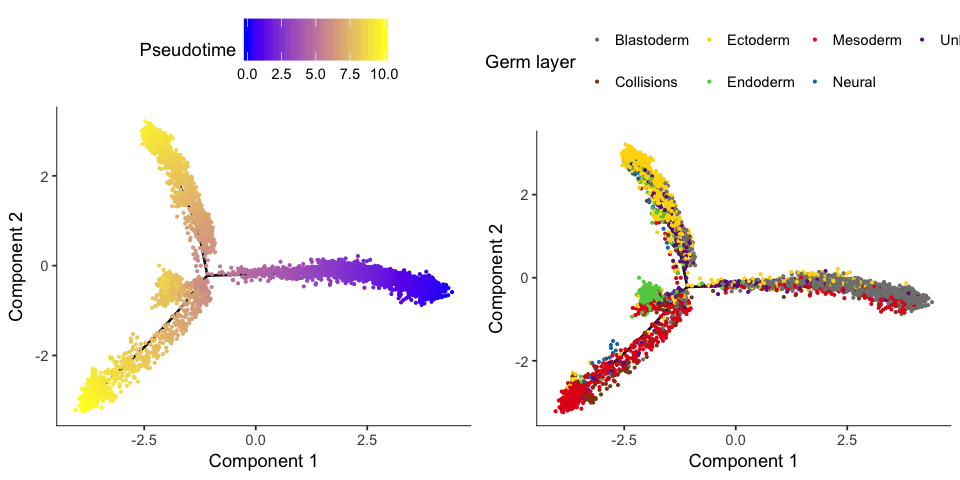For more examples of analyses that you can do with monocle, please visit the monocle website. If you’d like to interact with the processed data and check out browser tracks for the clusters of cells we’ve identified, please visit our companion site. Finally, if you would like, you can download the raw data here, and we have also made the code we used to process the raw data available on github.

` sessionInfo() `